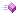This is the overview for the commaNum method overload.

 Name DescriptionAppend an integer, using commas to format the number (so 12345 would be appended as 12,345)Append an integer, using commas to format the number (so 12345 would be appended as 12,345)Append an integer, using commas to format the number (so 12345 would be appended as 12,345)Append an integer, using commas to format the number (so 12345 would be appended as 12,345)Append an integer, using commas to format the number (so 12345 would be appended as 12,345)